×#### Thank you for registering.

One of our academic counsellors will contact you within 1 working day.

Click to Chat

1800-1023-196

+91-120-4616500

CART 0

• 0

MY CART (5)

Use Coupon: CART20 and get 20% off on all online Study Material

ITEM
DETAILS
MRP
DISCOUNT
FINAL PRICE
Total Price: Rs.

There are no items in this cart.
Continue Shopping• Complete JEE Main/Advanced Course and Test Series
• OFFERED PRICE: Rs. 15,900
• View Details

```Revision Notes on Surface Areas and Volumes

Plane figure

The figures which we can draw on a piece of paper or which lie on a plane are called Plane Figures. Example - Circle, Square, Rectangle etc.

Solid figures

The 3D shapes which occupy some space are called Solid Figures. Example - Cube, Cuboid, Sphere etc.

Volume

Space occupied by any solid shape is the capacity or volume of that figure. The unit of volume is a cubic unit.

Surface Area

The area of all the faces of the solid shape is the total surface area of that figure. The unit of surface area is a square unit.

Lateral or Curved Surface Area

The surface area of the solid shape after leaving the top and bottom face of the figure is called the lateral surface of the shape. The unit of lateral surface area is a square unit.

Surface Area and Volume of a Cube

Cube is a solid shape having 6 equal square faces.Lateral surface area of a cube

4l2

Total surface area of a cube

6l2

The volume of a cube

l3

Diagonal

√3 l,  l = edge of the cube

Example

What is the capacity of a cubical vessel having each side of 8 cm?

Solution

Given side = 8 cm

So, Volume of the cubical vessel = l3

= (8)3 = 256 cm3.

Surface Area and volume of a Cuboid

Cuboid is a solid shape having 6 rectangular faces at a right angle.Lateral surface area of a cuboid

2h(l + b)

Total surface area of a cuboid

2(lb + bh + lh)

Volume of a cuboid

lbh

Diagonall = length, b = breadth, h = height

Example

What is the surface area of a cereal box whose length, breadth and height is 20 cm, 8 cm and 30 cm respectively?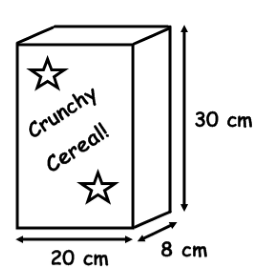Solution

Given,

Length = 20 cm

Height = 30 cm

Total surface area of the cereal box = 2(lb + bh + lh)

= 2(20 × 8 + 8 × 30 + 20 × 30)

= 2(160 + 240 + 600)

= 2(1000) = 2000 cm2.

Surface Area and Volume of a Right Circular Cylinder

If we fold a rectangular sheet with one side as its axis then it forms a cylinder. It is the curved surface of the cylinder. And if this curved surface is covered by two parallel circular bases then it forms a right circular cylinder.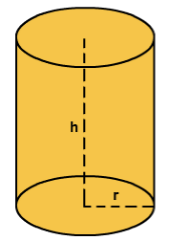Curved surface area of a Right circular cylinder

2πrh

Total surface area of a Right circular cylinder

2πr2 + 2πrh = 2πr(r + h)

The volume of a Right circular cylinder

πr2

r = radius, h = height

Surface Area and Volume of a Hollow Right Circular Cylinder

If a right circular cylinder is hollow from inside then it has different curved surface and volume.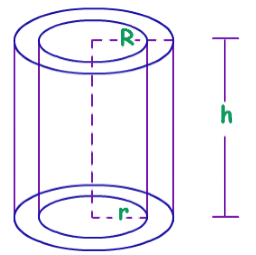Curved surface area of a Right circular cylinder

2πh (R + r)

Total surface area of a Right circular cylinder

2πh (R + r) + 2π(R2 - r2)

Example

Find the Total surface area of a hollow cylinder whose length is 22 cm and the external radius is 7 cm with 1 cm thickness. (π = 22/7)

Solution

Given, h = 22 cm

R = 7 cm

r = 6 cm (thickness of the wall is 1 cm)

Total surface area of a hollow cylinder = 2πh(R + r) + 2π(R2 - r2)

= 2(π) (22) (7+6) + 2(π)(72 - 62)

= 572 π + 26 π = 598 π

= 1878.67 cm2

Surface Area and Volume of a Right Circular Cone

If we revolve a right-angled triangle about one of its sides by taking other as its axis then the solid shape formed is known as a Right Circular Cone.Curved surface area of a Right Circular ConeTotal surface area of a Right Circular Cone

πr2 + πrl = πr(r + l)

The volume of Right Circular Cone

(1/3) πr2h

r = radius, h = height, l = slant height

Surface Area and Volume of a Sphere

A sphere is a solid shape which is completely round like a ball. It has the same curved and total surface area.Curved or Lateral surface area of a Sphere

4πr2

Total surface area of a Sphere

4πr2

Volume of a Sphere

(4/3) πr3

Surface Area and Volume of a Hemisphere

If we cut the sphere in two parts then is said to be a hemisphere.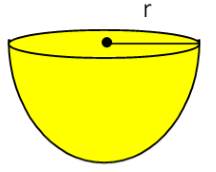Curved or Lateral surface area of a Sphere

2πr2

Total surface area of a Sphere

3πr2

Volume of a Sphere

(2/3) πr3

Example

If we have a metal piece of cone shape with volume 523.33 cm3 and we mould it in a sphere then what will be the surface area of that sphere?

Solution

Given, volume of cone = 523.33 cm3

Volume of cone = Volume of Sphere

Volume of sphere = 100 π cm3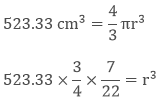125 = r3

r = 5

Surface area of a sphere = 4πr2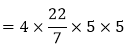= 314.28 cm2.
```### Course Features

• 728 Video Lectures
• Revision Notes
• Previous Year Papers
• Mind Map
• Study Planner
• NCERT Solutions
• Discussion Forum
• Test paper with Video Solution